Friday, April 3, 2009

PMML - Data Pre-Processing Primer

PMML defines several kinds of data transformations. These are:
• Normalization: map values to numbers, the input can be continuous (element NormContinuous) or discrete (element NormDiscrete).
• Discretization: map continuous values to discrete values.
• Value Mapping: map discrete values to discrete values.
• Text Indexing (text mining element introduced in PMML 4.2): derive a frequency-based value for a given term (not covered in this primer).
• Functions: derive a value by applying a function to one or more parameters.Below we will see how these transformations can be used and combined to allow for data powerful pre-processing in PMML and the Zementis products: ADAPA for real-time scoring and UPPI for Big Data scoring.

PMML transformations and much more are covered in the PMML book "PMML in Action" available on Amazon.com. Companion to the book and a learning tool for data pre-processing in PMML is the Transformations Generator, which is a graphical interface for learning PMML transformations.

Normalization: NormContinuous

This is a general method to normalize a continuous variable to another continuous variable. In the PMML code below, two normalizations take place and two derived variables are created. The first derived variable is named "DerivedNormalizedVar1" and the second "DerivedNormalizedVar2". The normalization itself is defined under the element NormContinuous. Typically, NormContinuous is used to normalize input values between 0 and 1 as shown in the first normalization example. However, as shown in the second normalization example, one can have as many LinearNorm elements as necessary and these do not need to normalized values between 0 and 1; the only restriction is that the first attribute orig must be in increasing order. The normalized variable is then a linearly interpolated value between the LinearNorm elements.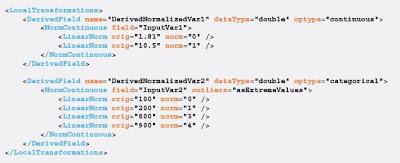Note that in the second example, we are using the attribute outliers to specify that any outliers to the normalization should be treated as extreme values. In this case, all "InputVar2" values lower than 100 will be assigned value "0" and all values greater than 900 will be assigned value "4".

Normalization: NormDiscrete

This method is used to transform string values to numeric values. Many models encode string values into numeric values in order to perform mathematical functions. For example, regression and neural network models often split categorical and ordinal variables into multiple dummy
variables.

The PMML code below implements the following logic:

IF CategoricalInputVar1 == Partner
THEN
DerivedVar1 = 1
ELSE
DerivedVar1 = 0

IF CategoricalInputVar1 == Associate
THEN
DerivedVar2 = 1
ELSE
DerivedVar2 = 0

IF CategoricalInputVar1 == Colleague
THEN
DerivedVar1 = 1
ELSE
DerivedVar1 = 0

In this example, variable "CategoricalInputVar1" has three possible valid values: "Partner", "Associate", and "Colleague". By using the NormDiscrete element, we can create derived variables (the dummy variables in this case) that will be assigned values "1" or "0" depending on the value of the categorical input variable being processed.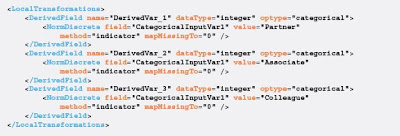Note that we are also handling missing values in this example. The mapMissingTo attribute means that if the input value "CategoricalInputVar1" is missing then all three derived variables will be assigned "0".

Discretization

This method maps continuous values to discrete string values. For a continuous variable we define intervals and if the variable falls inside that interval, we assign to it the string value defined for that interval. In the PMML code below, the Discretize element defines the continuous field "InputVar" which is to be discretized. The DiscretizeBin elements define the intervals. The first one defines a bin which has a rightMargin of 1000. By default, its left margin (attribute leftMargin) is negative infinity. The closure attribute finally defines the interval as (-infinity,1000], i.e. the right margin is less than or equal to 1000. So if the value of the variable "InputVar" falls in this interval, we assign to the derived variable "DerivedVar" the string value “low”. Similarly, if the value of "InputVar" is in (1000,100000], the derived value is equal to "medium" and if it is in (100000,1000000), the derived value is equal to "high".The mapping from a continuous to a discrete variable can be many-to-one but not one-to-many. In other words, two intervals can be mapped to the same string value but the same interval may not have more than one string value. This implies that the intervals defined must be disjoint; there can be no overlap between two defined intervals.

One can establish what should happen if the input is not in any of the defined intervals. The attribute defaultValue in the Discretize element establishes that if the input does not lie in any of the intervals (as for example, if "InputVar"=2,000,000), then by default it is assigned the value "extreme".

Discretization can also be paired up with element NormDiscrete to generate dummy variables which indicate if an input variable belongs to a certain interval. The PMML example shown below implements just that. We assume a numeric input variable named "InputVar" which leads to the creation of three dummy variables: "DerivedVar1", "DerivedVar2", and "DerivedVar3". These are used to indicate membership of "InputVar" to one of the following intervals: (-infinity,100], (100,200], and (200,+infinity).Note that in this example, we are using the Discretize element to map continuous values to an integer which is then used by the NormDiscrete element to indicate interval membership.

Value Mapping

This method is used to map discrete values to discrete values. This is done by using a table which lists the input values and the mapped output values. Each row in the table refers to a possible value for the input variable. Each column in the table has a name which is used to refer to that column. This is defined inside the element MapValues.

In the PMML code below, the attribute outputColumn in element MapValues establishes that the mapped output value will be found in the column named "color". The element FieldColumnPair uses the attribute field to define the input for mapping which consists of one or more input variables. In this example, we use two variables "InputVar1" and "InputVar2". We find the input value in the "animal" column for "InputVar1" and the input value in the "property" column for "InputVar2" and map them to the output value in the"color" column.The InlineTable element finally defines the table to be used for value mapping. So for example, if variable "InputVar1" has the value "dog" and variable "InputVar2" has the value "smart", we find "dog" in column "animal" and "smart" in column "property", both in the first row. These are them mapped to the value in the column named "color" in the same row. In this way, derived field "DerivedVar" is assigned the value "red".

As in the Discretize element, the MapValues element can have a defaultValue attribute which specifies what to map the input to if it does not have a matching value in any row.

In the PMML code below, we use elements MapValues and NormDiscrete to group small sets of categorical values. More specifically, we want to find out if input variable "VarInputColor" belongs to a specific group of colors. We do that by using the InLineTable element of MapValues to map different colors to the same number. We then use the element NormDiscrete to create dummy variables which are used to indicate group membership. This problem can be represented schematically in the following way:

IF VarInputColor is in ("Yellow","Red")
THEN
VarColorGroup_1 = 1
ELSE
VarColorGroup_1 = 0

IF VarInputColor is in ("Blue","Green")
THEN
VarColorGroup_2 = 1
ELSE
VarColorGroup_2 = 0Note that in the example shown above we are mapping missing values to value"2" and setting the default value to "1" inside the MapValues transformation element. Derived field "GroupedInputColor" is assigned the result of the mapping between the input strings and the number representing the group. This variable is then used to generate the desired output. If "GroupedInputColor" contains value "1", variable "VarColorGroup_1" is assigned value "1", otherwise it is assigned value "0". If "GroupedInputColor" contains value "2", variable "VarColorGroup_2" is assigned value "1", otherwise it is assigned value "0". This is accomplished by the use of the NormDiscrete element as described earlier.

Large sets of values can be handled in ADAPA by an external mapping table. For example, imagine that you would like to map all existing colors (that you can imagine) into four different groups and then create dummy variables to indicate such grouping. You can design this table externally (ADAPA allows external tables to be referred from a PMML file) and use the PMML element TableLocator to reference it from inside the MapValues transformation element. The PMML code below implements this functionality.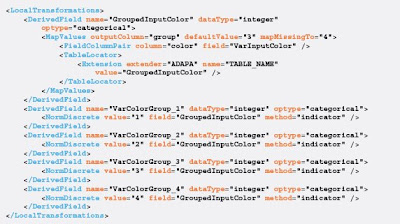Note that we are mapping missing values to value "4" and setting the default value to "3" inside the MapValues transformation element. Derived field "GroupedInputColor" is assigned the result of the mapping between the input colors and the number representing the group they belong to. As before, variable "GroupedInputColor" is then used to generate the desired output through the use of the element NormDiscrete.

The mapping table itself is only being referenced inside the element TableLocator. We are using a PMML extension in this case to indicate to ADAPA that the mapping table we want to access is named "GroupedInputColor".

Functions

If a certain transformation is to be applied to input data many times and to multiple fields, it makes sense to encapsulate the transformation inside a function and just use is as many times as necessary. This reduces the complexity of the PMML model and greatly simplifies its application. PMML provides a number of built-in functions as well as providing the capability for the user to define a user-defined function.

Built-in Functions

ADAPA supports all PMML built-in functions. The complete list is shown below.
• +, -, * and /
• min, max, sum, avg, median, product
• log10, ln, sqrt, abs, exp, pow, threshold, floor, ceil, round
• isMising, isNotMissing
• equal, notEqual, lessThan, lessOrEqual, greaterThan, greaterOrEqual
• and,or
• not
• concat
• matches, replace (regular expressions)
• uppercase
• substring
• trimBlanks
• formatNumber
• formatDatetime
• dateDaysSinceYear
• dateSecondsSinceYear
• dateSecondsSinceMidnight
Note that functions such as "min", "max", "sum" and "avg" take a variable number of parameters (derived fields or input fields) and return a single value which can then be assigned to a new derived field. Please refer to the DMG website - Built-in Functions for code examples and descriptions.

The PMML code shown below implements the following arithmetic operation:
ResultVar=maximum(round(InputVar1/3.3),2^(1+log(1.3*InputVar2+1)))

Note that it uses two different input variables: "InputVar1" and "InputVar2" as well as a number of numeric constants. The example shows many built-in functions which are used together to implement such a complex operation. The end result is assigned to derived variable "ResultVar".PMML also defines many functions that support boolean operations. These are used to compare parameters which are required to be of identical type (e.g., strings or dates) or of compatible type for numeric variables (e.g., double vs. integer). The result is of boolean type: "true" or "false", which is evaluated by functions such as "if" and "not".

PMML functions which operate on two input attributes, e.g. "a" and "b", of identical or compatible types are:
• equal: evaluates to "true" if "a" is equal to "b", "false" otherwise.
• notEqual: evaluates to "true" if "a" is not equal to "b", "false" otherwise.
• lessThan: evaluates to "true" if "a" is less than "b", "false" otherwise.
• lessOrEqual: evaluates to "true" if "a" is less or equal to "b", "false" otherwise.
• greaterThan: evaluates to "true" if "a" is greater than "b", "false" otherwise.
• greaterOrEqual: evaluates to "true" if "a" is greater or equal to "b", "false" otherwise.
• isIn: evaluates to "true" if "a" is in "b", "false" otherwise. Attribute "b" in this case is an array of values.
• isNotIn: evaluates to "true" if "a" is not in "b", "false" otherwise. Attribute "b" in this case is an array of values.
PMML functions which operate on a single input attribute, e.g. "a", are:
• isMissing: evaluates to true if "a" is missing, i.e. equal to NULL, "false" otherwise.
• isNotMissing: evaluates to "true" if "a" is not missing, "false" otherwise.

PMML functions which evaluate boolean operations are:
• not: operates on a single boolean attribute. Negates existing boolean evaluation result.
• and: summarizes the results of two or more independent boolean operations. Evaluates to "true" only if all operations are "true", "false" otherwise.
• or: summarizes the results of two or more independent boolean operations. Evaluates to "true" if a single operation is "true", "false" only if all operations are "false".
• if: implements IF-THEN-ELSE logic. The ELSE part is optional.

Below, we give a few examples of how these functions can be used to implement logical operations. We start with the PMML code below which implements the following logical and arithmetic operations:

IF InputVar1 == "Partner"
THEN
DerivedVar1 = "P"
ELSE
DerivedVar2 = 2 * InputVar2

In this example, we are using "InputVar1" which contains a string to assign values to two very different derived variables: "DerivedVar1" which is a string and "DerivedVar2" which is an integer.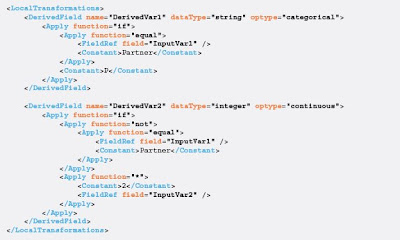Note that the code uses functions: "if", "equal", and "not" as well as the built-in fuction "*". The main reason for not using the "else" part of the "if" function is simply because we want to assign the "then" result to "DerivedVar1" and the "else" result to a different variable, "DerivedVar2". Data transformations in PMML are encapsulated under a single DerivedField element.

The PMML code below assumes that both "then" and "else" parts of the "if" use the same derived variable "DerivedVar1" to implement the following operations:

IF InputVar1 == "Partner"
THEN
DerivedVar1 = "5.1 * InputVar2"
ELSE
DerivedVar1 = "InputVar2 / 3.3"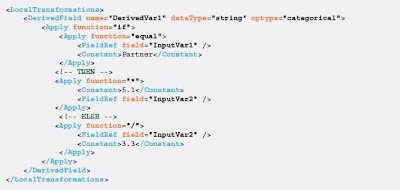Note that in this case, "DerivedVar2" is not being used. The "then" and "else" part are being used to assign the same variable "DerivedVar1" the result of two different computations.

We showed earlier how to use the Discretize element in conjunction with NormDiscrete to create dummy variables to indicate membership to numeric intervals. What if we would like to do just that, but this time for strings? The PMML code below exemplifies how this could be accomplished by using functions "if" and "lessOrEqual" in conjunction with the NormDiscrete element.

The PMML code below implements the following operations:

IF InputVar less or equal to "Denmark"
THEN
DerivedVar1 = 1 (otherwise = 0)
ELSE
IF InputVar less or equal to "France"
THEN
DerivedVar2 = 1 (otherwise = 0)
ELSE
DerivedVar3 = 1 (otherwise = 0)Note that the three dummy variables: "DerivedVar1", "DerivedVar2", and "DerivedVar3" are used to indicate the membership of input variable "InputVar" to three different string intervals.

Finally, we end our list of PMML code examples by showing the use of functions "isMissing" and "isIn" combined with function "if". The example shown in implements the following operations:

IF InputVar is missing
THEN
DerivedVar = 1
ELSE
IF InputVar is in ("Partner", "Associate", "Colleague")
THEN
DerivedVar = 2
ELSE
DerivedVar = 3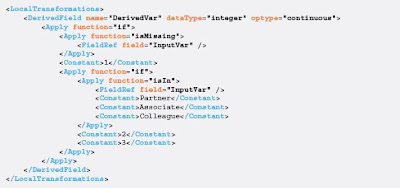When defining a PMML document, the pre-processing of the input variables is mainly located inside the following PMML elements: TransformationDictionary and LocalTransformations. Although the TransformationDictionary element is mostly used for user-defined functions (element DefineFunction).

For the formal PMML schema definition of the transformations covered here, please refer to the PMML Transformations page on the DMG website.

1.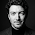I just want computers to make sense!

2.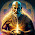I am curious, and feeling bit rebel.
Why XML, why any specific data defining flat file schema and markup.
We have progressed so far, and still working with boundaries of any markup??
Sorry, for bit rebellious on your post, which is definitely a stepping stone for many others to come, so, my my comment may do some reach.
I agree with Villads Claes, but in linguistic terms. Multiple reasons,
1) Why We are with in language barriers, in computer science. This post is in English, Markup and definition is in English. Brackets which are used are from english.
2) Why not diagrams ? Why not some circutry.. plain vannila.. photographs. Will that will not make everyone happy ?
3) Why XML ? another debate, and why markup ? Why not JSON/YAML/TOML/Binary or any other format.. as thing should be predictive. Right. XML just because of DTD or XSD being predictive which is another markup..
4) I want my friends, who are mathematicians, for them, to do their job best with out learning a new language, they can use their own universal language which is numbers. Isn't computer language / markup or this innovation comes from mathematics.. and who say mathematics can't be diagrammatic..

I am totally aware, people or moderator who are going to be very furious, after reading this.
But guys, may be wrong place, but you can help us out to reach right message with right peoples.